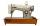# Cupcakes 2

Susi has 25 cupcakes. She gives 4/5. How much does she have left?

Result

n =  20

#### Solution:Leave us a comment of example and its solution (i.e. if it is still somewhat unclear...):

Showing 0 comments:Be the first to comment!#### To solve this example are needed these knowledge from mathematics:

Need help calculate sum, simplify or multiply fractions? Try our fraction calculator.

## Next similar examples:

1. TeacherTeacher Rem bought 360 pieces of cupcakes for the outreach program of their school. 5/9 of the cupcakes were chocolate flavor and 1/4 wete pandan flavor and the rest were vanilla flavor. How much more chocolate flavor cupcakes than vanilla flavor?
2. Pizza 4Marcus ate half pizza on monday night. He than ate one third of the remaining pizza on Tuesday. Which of the following expressions show how much pizza marcus ate in total?
3. Bitoo and ReenaBitoo ate 3/5 part of an apple and the remaining part was eaten by his sister Reena. How much part of an apple did Renna eat? Who had the larger share? By how much?
4. In fractionsAn ant climbs 2/5 of the pole on the first hour and climbs 1/4 of the pole on the next hour. What part of the pole does the ant climb in two hours?
5. WithdrawalIf I withdrew 2/5 of my total savings and spent 7/10 of that amount. What fraction do I have in left in my savings?
6. ZdeněkZdeněk picked up 15 l of water from a 100-liter full-water barrel. Write a fraction of what part of Zdeněk's water he picked.
7. Fraction and a decimalWrite as a fraction and a decimal. One and two plus three and five hundredths
8. Lengths of the poolMiguel swam 6 lengths of the pool. Mat swam 3 times as far as Miguel. Lionel swam 1/3 as far as Miguel. How many lengths did mat swim?
9. Passenger boatTwo-fifths of the passengers in the passenger boat were boys. 1/3 of them were girls and the rest were adult. If there were 60 passengers in the boat, how many more boys than adult were there?
10. Fraction to decimalWrite the fraction 3/22 as a decimal.
11. Write 2Write 791 thousandths as fraction in expanded form.
12. Mixed2improperWrite the mixed number as an improper fraction. 166 2/3
13. Adding mixed numerals3 3/4 + 2 3/5 + 5 1/2 Show your solution.
14. TailorTailor bought 2 3/4 meters of textile and paid 638 CZK. Determine the price per 1 m of the textile.
15. Trees3/5 trees are apples, cherries are 1/3. 5 trees are pear. How many is the total number of trees?
16. Reciprocal equation 3Solve reciprocal equation: 1/2 + 2/3=1/x
17. Sum of fractionsWhat is the sum of 2/3+3/5?